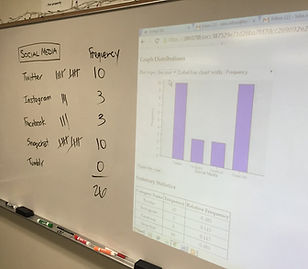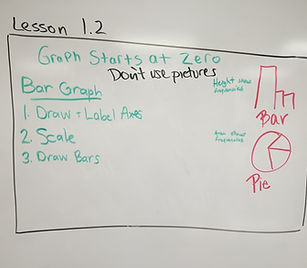## Day 4 - Lesson 1.2

##### Learning Targets
• Make and interpret bar charts of categorical data.

• Interpret bar charts.

• Identify what makes some graphs of categorical data deceptive.

##### Homework

We decided that we are going to give less homework problems with the hopes that students recognize the value of homework and work on it authentically.  The magic number we settled on is 5.  Only 5 homework problems.  Typically the first 2 or 3 will directly address the learning targets, the next 2 will be problems where they need to put it all together, and then 1 review problem (read Make It Stick if you want to know why – interleaving learning).  This means that at the start of each day, we often will only go over 1 homework problem.

##### Activity:

We are going to try to have some sort of activity to start each new lesson.  We use the word “Activity” loosely, but as a general rule means that students are doing something within a real context.  Today, we asked “What is the most used social media app by students?”  Students collected the data for the class and displayed it in a frequency table and a relative frequency table (so we can connect new learning to previous learning).  Then we used iPads to make bar charts and pie graphs. We are going to use the applets found here for several activities in Chapter 1.So we are going to try to run this whole class with no calculators….just iPads.  Today I have students working with one iPad for each pair of students.  This allows students to help each other if they are not familiar with the use of an iPad.  I demonstrated the use of the iPad by beaming my iPad to the projector using an Apple TV.  This process is not yet seamless but will hopefully getting better.

##### Going Through the Text and then Applications

Again I modeled going through the text with highlighting and annotating.  Students worked again in groups of 4 to pick out the major ideas of the lesson.   Then we worked on a few example problems to make sure that they could apply their new learning.